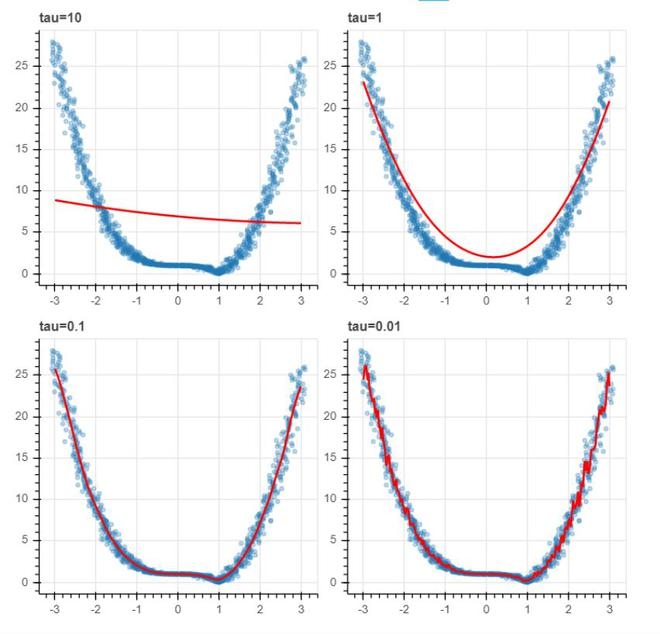Related Articles

# Locally weighted linear Regression using Python

• Last Updated : 18 Jul, 2021

Locally weighted linear regression is the nonparametric regression methods that combine k-nearest neighbor based machine learning. It is referred to as locally weighted because for a query point the function is approximated on the basis of data near that and weighted because the contribution is weighted by its distance from the query point.

Locally Weighted Regression (LWR) is a non-parametric, memory-based algorithm, which means it explicitly retains training data and used it for every time a prediction is made.

To explain the locally weighted linear regression, we first need to understand the linear regression. The linear regression can be explained with the following equations:Let (xi, yi) be the query point, then for minimizing the cost function in the linear regression:by calculatingso, that it minimize the above cost function.
Our output will be:Thus, the formula for calculating \theta can also be:where, beta is the vector of linear vector, X, Y is the matrix, and vector of all observations.

For locally weighted linear regression:by calculating
so, that it minimize the above cost function.
Our output will be:Here, w(i) is the weight associated with each observation of training data. It can be calculated by the given formula:Or this can be represented in the form of a matrix calculation:Impact of Bandwidth

where x(i) is the observation from the training data and x is a particular point from which the distance is calculated and T(tau) is the bandwidth. Here, T(tau) decides the amount of fitness in the function, if the function is closely fitted, its value will be small. Therefore,then, we can calculate \theta with the following equation:### Implementation

• For this implementation, we will be using bokeh. If you want to know bokeh functionalities in details please check this article

## Python3

 # Necessary importsimport numpy as npfrom ipywidgets import interactfrom bokeh.plotting import figure, show, output_notebookfrom bokeh.layouts import gridplot%matplotlib inlineoutput_notebook()plt.style.use('seaborn-dark')  # function to perform lcally weighted linear regressiondef local_weighted_regression(x0, X, Y, tau):    # add bias term    x0 = np.r_[1, x0]    X = np.c_[np.ones(len(X)), X]          # fit model: normal equations with kernel    xw = X.T * weights_calculate(x0, X, tau)    theta = np.linalg.pinv(xw @ X) @ xw @ Y    # "@" is used to    # predict value    return x0 @ theta  # function to perform weight calculationdef weights_calculate(x0, X, tau):    return np.exp(np.sum((X - x0) ** 2, axis=1) / (-2 * (tau **2) ))  # plot locally weighted regression for different bandwidth valuesdef plot_lwr(tau):    # prediction    domain = np.linspace(-3, 3, num=300)    prediction = [local_regression(x0, X, Y, tau) for x0 in domain]      plot = figure(plot_width=400, plot_height=400)    plot.title.text = 'tau=%g' % tau    plot.scatter(X, Y, alpha=.3)    plot.line(domain, prediction, line_width=2, color='red')          return plot  #define distributionn = 1000  # generate datasetX = np.linspace(-3, 3, num=n)Y = np.abs(X ** 3 - 1)  # jitter XX += np.random.normal(scale=.1, size=n)  # show the plots for different values of Taushow(gridplot([    [plot_lwr(10.), plot_lwr(1.)],    [plot_lwr(0.1), plot_lwr(0.01)]]))LWR plot with different values of bandwidth

• As we can notice from the above plot that with small values of bandwidth, the model fits better but sometimes it will lead to overfitting.

### References:

Attention reader! Don’t stop learning now. Get hold of all the important Machine Learning Concepts with the Machine Learning Foundation Course at a student-friendly price and become industry ready.

My Personal Notes arrow_drop_up